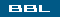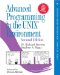# Section 14.8. readn and writen Functions### 14.8. readn and writen Functions

Pipes, FIFOs, and some devices, notably terminals, networks, and STREAMS devices, have the following two properties.

1. A read operation may return less than asked for, even though we have not encountered the end of file. This is not an error, and we should simply continue reading from the device.

2. A write operation can also return less than we specified. This may be caused by flow control constraints by downstream modules, for example. Again, it's not an error, and we should continue writing the remainder of the data. (Normally, this short return from a write occurs only with a nonblocking descriptor or if a signal is caught.)

We'll never see this happen when reading or writing a disk file, except when the file system runs out of space or we hit our quota limit and we can't write all that we requested.

Generally, when we read from or write to a pipe, network device, or terminal, we need to take these characteristics into consideration. We can use the following two functions to read or write N bytes of data, letting these functions handle a possible return value that's less than requested. These two functions simply call read or write as many times as required to read or write the entire N bytes of data.

 ` #include "apue.h" ssize_t readn(int filedes, void *buf, size_t nbytes); ssize_t writen(int filedes, void *buf, size_t nbytes);` Both return: number of bytes read or written, 1 on error

We define these functions as a convenience for later examples, similar to the error-handling routines used in many of the examples in this text. The readn and writen functions are not part of any standard.

We call writen whenever we're writing to one of the file types that we mentioned, but we call readn only when we know ahead of time that we will be receiving a certain number of bytes. Figure 14.29 shows implementations of readn and writen that we will use in later examples.

##### Figure 14.29. The readn and writen functions
` #include "apue.h" ssize_t             /* Read "n" bytes from a descriptor */ readn(int fd, void *ptr, size_t n) {     size_t       nleft;     ssize_t      nread;     nleft = n;     while (nleft > 0) {         if ((nread = read(fd, ptr, nleft)) < 0) {             if (nleft == n)                 return(-1); /* error, return -1 */             else                 break;      /* error, return amount read so far */         } else if (nread == 0) {             break;          /* EOF */         }         nleft -= nread;         ptr += nread;     }     return(n - nleft);      /* return >= 0 */ } ssize_t             /* Write "n" bytes to a descriptor */ writen(int fd, const void *ptr, size_t n) {     size_t      nleft;     ssize_t     nwritten;     nleft = n;     while (nleft > 0) {         if ((nwritten = write(fd, ptr, nleft)) < 0) {             if (nleft == n)                 return(-1); /* error, return -1 */             else                 break;      /* error, return amount written so far */         } else if (nwritten == 0) {             break;         }         nleft -= nwritten;         ptr   += nwritten;     }     return(n - nleft);      /* return >= 0 */ } `

Note that if we encounter an error and have previously read or written any data, we return the amount of data transferred instead of the error. Similarly, if we reach end of file while reading, we return the number of bytes copied to the caller's buffer if we already read some data successfully and have not yet satisfied the amount requested.Advanced Programming in the UNIX Environment, Second Edition (Addison-Wesley Professional Computing Series)
ISBN: 0321525949
EAN: 2147483647
Year: 2005
Pages: 370

Similar book on Amazon